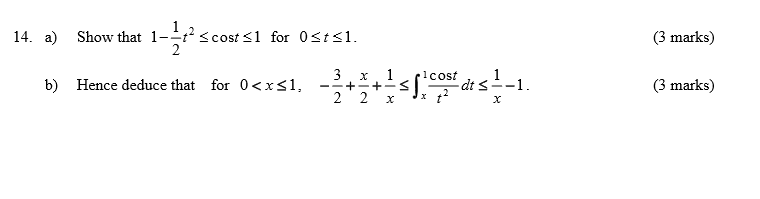# Trigonometry inequality

## Homework Statement

show that 1-t^2/2 <=cos(t) <=1 for 0<=t<=1

## Homework Equations

Trigonometry knowledge

## The Attempt at a Solution

I don't know how to relate t with cos(t), and I also try to find out cos(1), but there is no result, so how can I start with this problem.

SammyS
Staff Emeritus
Science Advisor
Homework Helper
Gold Member

## Homework Statement

show that 1-t^2/2 <=cos(t) <=1 for 0<=t<=1

## Homework Equations

Trigonometry knowledge

## The Attempt at a Solution

I don't know how to relate t with cos(t), and I also try to find out cos(1), but there is no result, so how can I start with this problem.
Are you sure that it's 0 ≤ t ≤ 1 , and not 0 ≤ t ≤ π/2 ?

Yes, this is what the question said. How can you solve for 0 <= t <= pi/2 ?Mark44
Mentor
show that 1-t^2/2 <=cos(t) <=1 for 0<=t<=1
Have you know about Maclaurin series yet, and in particular, the Maclaurin series for cos(t)?

From another question you posted, you are taking a calculus class. If so, both questions should have been posted in the Calculus & Beyond section, not the Precalculus section.